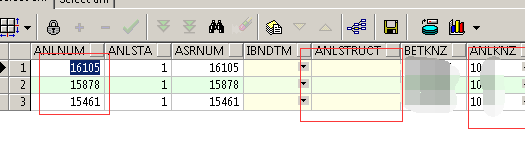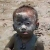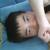## oracle存储过程

就是我想写一个存储过程，anlknz(有2、5、7、9位）2位数字 为10，20，30，5为的前面两位是2位的数字，anlnum是主键、anlstruct是结构，如果5为数字前两位是一样的就把两位数字的主键放到anlstruct字段中，怎么写啊00anlknz里的数字两位的为10 ，20，30 那么五位的为10 111 或者20 111 或者 30 111 七位的 为10 111 12 一次类推
0#### 引用来自“LinuxGod”的评论

```select f_gzdycode,
decode(substr(f_gzdycode, 3, 1),
substr(f_gzdycode, 4, 1),
'相等',
'不相等')
from t_busi_gzdy_tmccl_xz xz
where f_gzdycode is not null;```

0

#### 引用来自“LinuxGod”的评论

```select f_gzdycode,
decode(substr(f_gzdycode, 3, 1),
substr(f_gzdycode, 4, 1),
'相等',
'不相等')
from t_busi_gzdy_tmccl_xz xz
where f_gzdycode is not null;```

DECLARE
CURSOR anl_cur IS
SELECT * FROM anl FOR UPDATE;
length1 number;
BEGIN
open anl_cur;
fetch anl_cur

into length1 when anl_cur%FOUND;

LOOP
IF length1 = 2 THEN
update anl a
set a.anlstruct =
(select anlnum
from anl
where length(anlknz) = 2
and anlknz in (select substr(anlknz, 1, 2)
from anl
where length(anlknz) = 5))
where length(a.anlknz) = 5
and (select anlknz from anl where length(anlknz) = 2) in
(select substr(anlknz, 1, 2) from anl where length(anlknz) = 5);

END IF;
END LOOP;
close anl_cur;
END;
ORA-06550: 第 8 行, 第 18 列:
PLS-00103: 出现符号 "WHEN"在需要下列之一时：
. ( , % ; limit
ORA-06550: 第 9 行, 第 3 列:

PLS-00103: 出现符号 "LOOP"

0ablknz输入
```10
10 111
10 111 22
10 111 22 33```

```10 111，anlstruct设置为10
10 111 22，anlstruct设置为10 111
10 111 22 33，anlstruct设置为10 111 22```

```update t a set anlstruct=(select b.anlnum from t b where
(length(a.ablknz)>5 and substr(a.ablknz,1,length(a.ablknz)-2)=b.ablknz )
or
(length(a.ablknz)=5 and substr(a.ablknz,1,2)=b.ablknz)
)
where exists(select null from t b where
(length(a.ablknz)>5 and substr(a.ablknz,1,length(a.ablknz)-2)=b.ablknz )
or
(length(a.ablknz)=5 and substr(a.ablknz,1,2)=b.ablknz)
)```

0ablknz输入
```10
10 111
10 111 22
10 111 22 33```

```10 111，anlstruct设置为10
10 111 22，anlstruct设置为10
10 111 22 33，anlstruct设置为10```

```update t a set anlstruct=(select b.anlnum from t b where
substr(a.ablknz,1,2)=b.ablknz
)
where exists(select null from t b where
substr(a.ablknz,1,2)=b.ablknz
)```